THEOREM 1
THEOREM 2
THEOREM 3
THEOREM 4
TERMS
100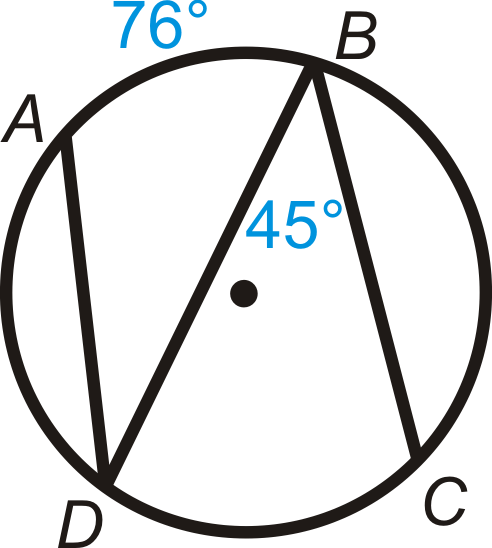Find mDC

90

100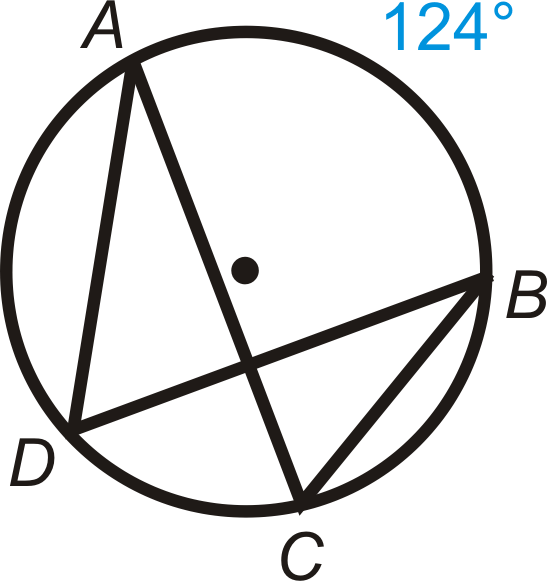62

100

Find m∠DAB in ⨀C.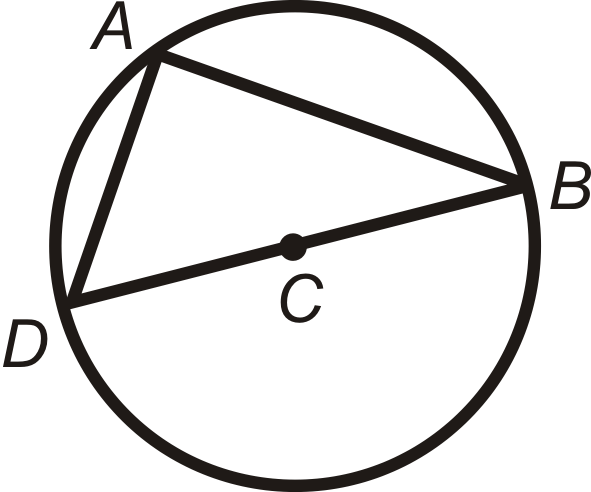90

100

Find the measures of the angle a. Use the figure on the right.m<a = 108

100

An arc measuring one-half the circumference of a circle.

SEMICIRCLE

20038

200

Find m∠ACB.62.

200

A line segment with one endpoint in the center of the circle and one point on the circle.

200

This is the relationship between opposite angles of a quadrilateral inscribed in a circle.

They are Supplementary

200

An angle formed by two rays whose vertex is the center of the circle.

CENTRAL ANGLE

300

Find the value of x in ⨀A.

1.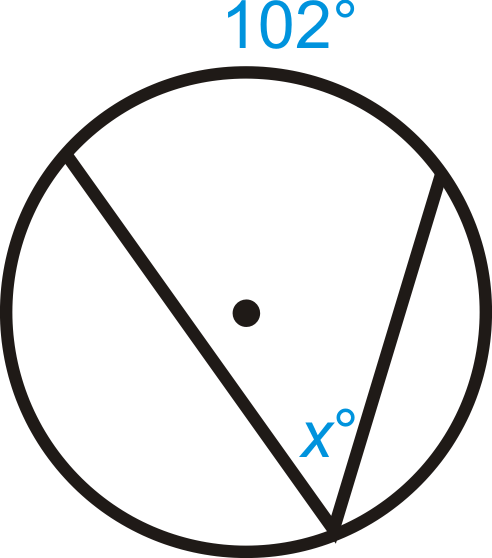51

300

This is the relationship between two adjacent arcs that cover the entire circle.

What is sum to 360 degrees?

300

This is the size of the angle inscribed in a semicircle.

right angle

300

Two chords are each measured as 36 centimeters long. One chord is 16 centimeters from the center. This is the distance the second chord is from the center.

What is 16 centimeters?

300

An angle whose vertex is on a circle and whose sides contain chords of the circle.

INSCRIBED ANGLE

400

Find the value of x in ⨀A.

1.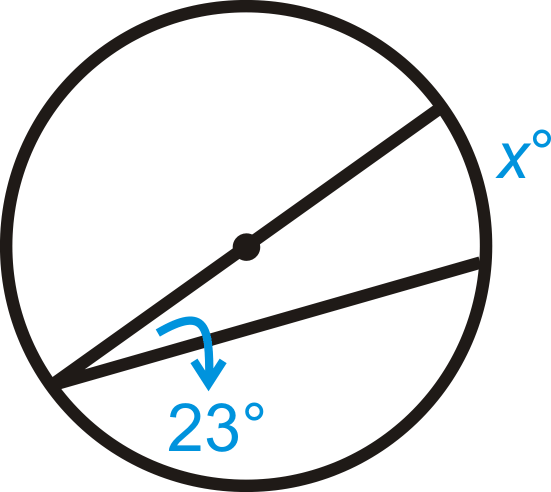46

400

Solve for x.

1.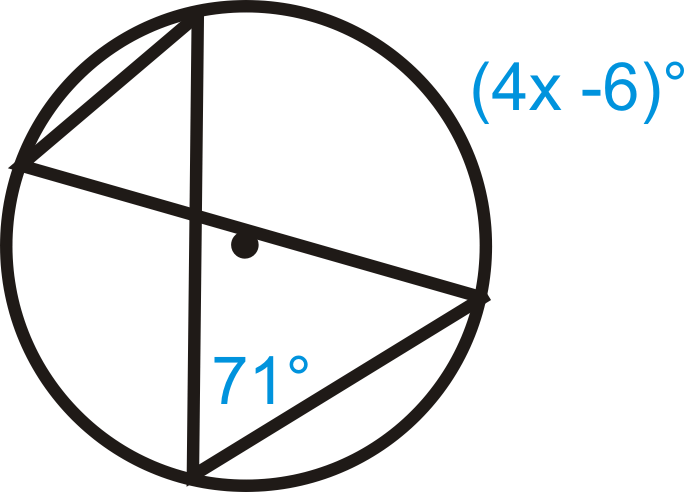x = 37

400

An angle with the vertex on the circle, formed by two chords.

What is an inscribed angle?

400

The sides of an inscribed angle are ___________________.

chords

400

An arc that lies in the interior of an inscribed angle and has endpoints of the angle.

INTERCEPTED ARC

500

This is the relationship between opposite angles of a quadrilateral inscribed in a circle.

1/2 arc = angle?

500

This is the relationship between central angles and the corresponding arc formed by the radii.

What is congruent?

500

Solve for x.

1.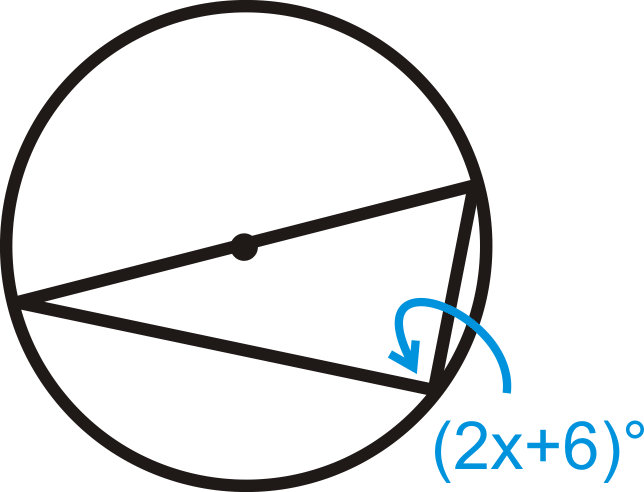x = 42

500

If a chord, measuring 20 inches, is bisected by a line segment starting at the center and the radius is 26 inches, this is the length of the line segment.

What is 24 inches?

500

State theorem 3 on inscribed angles.

If an inscribed angle of a circle intercepts a semicircle, then the angle is a right angle.

Click to zoom